Question

A vessel at rest at the origin of an xy coordinate system explodes into three pieces. Just after the explosion, one piece, of mass m, moves with velocity (-29 m/s) and a second piece, also of mass m, moves with velocity (-29 m/s) . The third piece has mass 3m. Just after the explosion, what are the (a) magnitude and (b) direction (as an angle relative to the +x axis) of the velocity of the third piece?

1.huyenthanh

We are given that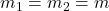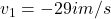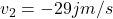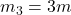a.Initial velocity of vessel,u=0

According to law of conservation of momentum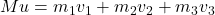Where M=Mass of  vessel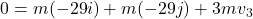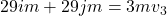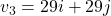m/s

Magnitude,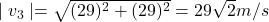b.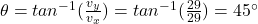Hence, direction of the velocity of third piece=45 degree